Home Basic Data Analysis Time Series Decomposition & Prediction in Python

# Time Series Decomposition & Prediction in Python

In this article I wanted to concentrate on some basic time series analysis, and on efforts to see if there is any simple way we can improve our prediction skills and abilities in order to produce more accurate results. When considering most financial asset price time series you would be forgiven for concluding that, at various time frames (some longer, some shorter) many, many of the data sets we try to analyse can appear completely random. At least random enough that any hope of easily forecasting future value and paths is going to be a tough ask at the every least!

Although there most probably isn’t an easy fix just around the corner, there are perhaps some tools available to us that could assist.

Time series decomposition is a technique that allows us to deconstruct a time series into its individual “component parts”. These parts consist of up to 4 different components:

1) Trend component2) Seasonal component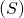3) Cyclical component4) Noise componentn.b we sometimes lump together the Cyclical and Noise components and call it the “Remainder” or some such.

A particular time series doesn’t need to contain all 4 components, it may be lacking a seasonal or trend component.

So what use is this knowledge to us? Well predicting a time series can often be really rather difficult but if we can decompose the series into components and treat each one separately we can sometimes improve overall prediction. Each component has specific properties and behaviour and with this approach we are able to use methods that are more suited to each particular component.

It is worth noting that decomposition is mainly used to help analyse and understand historical time series, but it can also prove useful when attempting a forward looking analysis.

So as mentioned previously, a series is made up of, and can be decomposed into, a number of components: Trend, Seasonality, Cyclicaland Noise– these last two are sometimes combined and labelled as the Remainder. It is helpful to think of the components as combining either additively or multiplicatively.

They differ as follows:

1) An additive model suggests that the components are added together as follows: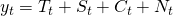whereas a multiplicative model suggests that the components are multiplied together as follows:2) An additive model is linear where changes over time are consistently made by the same amount, whereas a multiplicative model is nonlinear, such as quadratic or exponential and changes increase or decrease over time.

3) A linear trend is a straight line, whereas a nonlinear trend is a curved line.

4) A linear seasonality has the same frequency (width of cycles) and amplitude (height of cycles), whereas a non-linear seasonality has an increasing or decreasing frequency and/or amplitude over time.

Just as an point to note, the above is a general rule and in practice you may or may not be able to cleanly or perfectly break down your specific time series as an additive or multiplicative model. There may exists combinations of the two models, trends can change direction and cycles of a non-repeating nature can be mixed in with the repeating seasonality components. Basically real-world data can be messy and it doesn’t always play by the rules! They are still helpful though in defining a simple framework from which to analyse our data.

There are various methods of decomposition, with the “base” method known as “classical decomposition”, a relatively simple procedure that also forms the starting point for most other methods of time series decomposition. This method has been around for years, originating way back in the 1920s – so this isn’t exactly anything “new”.

There are two forms of classical decomposition, one for each of our two models described above (additive an multiplicative).

For additive decomposition the process (assuming a seasonal period of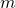) is carried out as follows:

1) Compute the “trend-cycle” component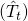using a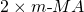ifis an even number, or using an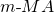ifis an odd number.

2) Calculate the detrended series:3) The seasonal component for each season if then calculated by simply averaging the detrended values for that season. So if we are dealing with monthly data, the seasonal component for December, for example, would just be the average of all the detrended December values in the data. Adjustments to these monthly values are made to ensure that they all sum to zero, and then they are strung together and the sequence is replicated for each year of data: the output of this is4) The final step is to calculate the noise component by subtracting the estimated “seasonal” and “trend-cycle” components:Classical multiplicative decomposition is similar, except that the subtractions are replaced by divisions.

While classical decomposition is still widely used, it is not recommended, as it suffers from multiple problems, such as having no trend-cycle estimates for the first few and last few observations (e.g. if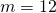there would be no trend-cycle estimate for the first ans last 6 time periods, and therefore no remainder component either). It also suffers from a few other weakness regarding the assumptions made that the seasonal component repeats from year to year, it tends to over-smooth rapid rises and falls in the data when calculating the trend-cycle and the method is also not robust to “unusual” or “outlier” values in the data. Anyway, there are now several much better methods available such as X11 Decomposition, SEATS Decomposition or STL Decomposition.

Let’s go with STL Decomposition; the “STL is an acronym for “Seasonal and Trend decomposition using Loess”. Loess is a method for estimating non-linear relationships.

STL has several advantages over the classical, SEATS and X11 decomposition methods:

1) STL will handle any kind of seasonality, not only monthly and quarterly (unlike SEATS and X11).

2) The smoothness of the trend-cycle can be controlled by the user

3) The seasonal component is allowed to change over time, again allowing the user to control this rate.

4) It is generally more robust to “unusual”, or outlier data points. It can be carried out in a way that the estimates of the trend-cycle and seasonal components will not be affected, however the remainder component will still be affected.

Just to be fair, it does also have some disadvantages, for example it is only able to be used with additive decomposition and it doesn’t automatically account for, and handle trading day/calendar variation.

OK let’s break up the wall of text and actually implement a quick decomposition using the statsmodels package. I will be using the EURUSD price time series in this instance and the relevant data can be downloaded below:

Now I should make it clean that the STL class used below has JUST been added to statsmodels about 10 days ago (its 21/07/2019 as I write this) so it is NOT available in the current stable version release that pip will install for you. To get this version you have to follow a couple of steps, but it’s still very easy to install and use. Firstly PLEASE USE A VIRTUAL ENVIRONMENT WHEN INSTALLING THE DEVELOPMENT VERSION!!! I don’t want to be responsible for causing any weird and wonderful problems regarding your current, main install – so yeah again – PLEASE install this in a virtual environment.

With that word of caution, if you wish to avail yourself of the STL class you can follow these steps:

1) Create and activate a virtual environment

2) install Cython in that virtual environment

pip install cython

3) install statsmodels development version

pip install git+https://github.com/statsmodels/statsmodels.git

Once that is successfully completed, we go straight into the main code by importing the necessary modules. We then read in the EURUSD data using Pandas, extract the relevant column and run it through both a Hodrick-Prescott Filter and an SYL decomposition separately. Just to note, the EURUSD file provided is “tab separated” rather than the more common “comma separated” hence we set sep="\t".

import pandas as pd
import statsmodels.api as sm
from statsmodels.tsa.seasonal import STL
from sklearn.metrics import mean_squared_error
from statsmodels.tsa.ar_model import AR
import matplotlib.pyplot as plt
import seaborn as sns
# include below line if you are using Jupyter Notebook
%matplotlib inline
# Set figure width to 12 and height to 9
plt.rcParams['figure.figsize'] = [12, 9]
df.index = pd.to_datetime(df.index)
df.sort_index(inplace=True)
df = df.resample('W').last()
series = df['Price']

The HP filter is a technique commonly used with macro-economic series that have a trend (long-term movements), business cycle and irregular parts (short-term fluctuations). It constructs the trend component by solving an optimisation problem. It aims to form the smoothest trend estimate that minimises the squared distances to the original series. In other words, it has to find equilibrium between the smoothness of the trend and its closeness to the original.

If you want to find out more about the details, you can do that here.

First the HP filter decomposition:

cycle, trend = sm.tsa.filters.hpfilter(series, 50)
fig, ax = plt.subplots(3,1)
ax.plot(series)
ax.set_title('Price')
ax.plot(trend)
ax.set_title('Trend')
ax.plot(cycle)
ax.set_title('Cycle')
plt.show()

And next the SYL decomposition :

result = STL(series).fit()
chart = result.plot()
plt.show()

Right, all looks good so far – we have our decomposed series, from top down: Raw Weekly Price, Trend, Season and Residual (a.k.a Remainder). So what now? Well what if we want to try to forecast the EURUSD price movements? We could start by applying a simple “persistence model”, this is the simplest kind of model which works by assigning the last observed value as the prediction for the following value, i.e. it just “persists” the last price forward. As you can see its not exactly a very sophisticated model, however its a start and it provides a baseline of performance for the problem that we can use for comparison with an auto regression model. We will score our model performance using the Root Mean Squared Error (RMSE).

The code below begins by creating a series of “1-period forward” predictions, just shifting the last price forward one week and comparing that value with the actual price that was seen at that time. It then uses the scikit-learn “mean_squared_error” function to calculate the MSE, which we then simply take the square root of to produce the RMSE. We receive a value of 0.013.

You will notice that the RMSE is only calculated for the period corresponding to a “testing dataset”, which in this case is the last 30% of the data. This allows us to compare performance with more sophisticated models that require “training” over a “training” dataset, before they can be used for prediction purposes, the performance of which we judge when carried out over a “testing” dataset.

This ensures that we only judge a models performance when it is used to predict parts of a time series that it didn’t have available at the time of optimising its parameters.

The mean squared error, or MSE, is calculated as the average of the squared forecast error values. The error values are in squared units of the predicted values and a mean squared error of zero indicates perfect prediction skills, or “no error” in effect.

The MSE can be transformed back into the original units of the predictions by taking the square root as mentioned above with an RMSE of zero again indicating perfect prediction skills.

Finally, we plot just the last few data points, allowing us to see more clearly how the predicted values relate to the actual values observed.

predictions = series.shift(1).dropna()
test_score = np.sqrt(mean_squared_error(series[int(len(series) * 0.7)+1:], predictions.iloc[int(len(series) * 0.7):]))
print('Test RMSE: %.5f' % test_score)
plt.plot(series.iloc[-25:], label='Price')
plt.plot(predictions[-25:], color='red', label='Prediction')
plt.legend()
plt.show()

It might also be useful to create a scatter plot of the predicted vs the actual weekly percentage change and see if there is any noticeable relationship. We do this below, followed by calculating the Mean Absolute Error (MAE) of the predictions (i.e. by how much, on average did our prediction differ from the observed value)

fig, ax = plt.subplots()
ax = sns.regplot(series.iloc[-int(len(series) * 0.3):].pct_change(),
predictions.iloc[-int(len(series) * 0.3):].pct_change(), )
plt.xlabel('Observations')
plt.ylabel('Predictions')
plt.title('EURUSD Observed vs Predicted Values')
ax.grid(True, which='both')
ax.axhline(y=0, color='#888888')
ax.axvline(x=0, color='#888888')
sns.despine(ax=ax, offset=0)
plt.xlim(-0.05, 0.05)
plt.ylim(-0.05, 0.05)
plt.show()
mae = round(abs(series.iloc[-int(len(series) * 0.3):].pct_change() - predictions.iloc[-int(len(series) * 0.3):].pct_change()).mean(),4)
print(f'The MAE is {mae}')

Looking at the above plot it seems as though there is no discernable relationship between our predictions and our observed values when considering the weekly percentage change in the EURUSD price. That’s not great at all!

If we quickly calculate the “hit rate” of how often we are able to correctly predict the direction of next week’s move in the EURUSD, we can see that it is almost exactly 50% (in fact its a little worse than that even) – no better than completely randomly guessing!

price_pred = pd.concat([series.iloc[-int(len(series) * 0.3):].pct_change(), predictions.iloc[-int(len(series) * 0.3):].pct_change()], axis=1)
price_pred.dropna(inplace=True)
price_pred.columns = ['Price', 'preds']
price_pred['hit'] = np.where(np.sign(price_pred['Price']) == np.sign(price_pred['preds']), 1, 0)
print(f"Hit rate: {round((price_pred['hit'].sum() / price_pred['hit'].count()) * 100,2)}%")
48.49%

Now that we have our “baseline” model score, we have something to compare subsequent models to. Let’s try our predictions using an autoregressive model, which is basically a linear regression model that uses lagged values of variables as subsequent input variables.

There is a decision to make as to what lag to use for our input variable – the simplest approach for now is to use the AR class found in statsmodels which automatically selects an appropriate lag using various statistical tests and proceeds to train a linear regression model.

The code below takes our EURUSD time series and splits it into a “training” and “testing” dataset and creates an empty list which we are going to use to store our predictions. This is because this time we are going to make our weekly predictions one by one, in a “walk-forward” manner.

We are going to iterate through a “for-loop” with the number of loops dictated by the length of our test data – each time through the loop we are going to create our AR model and feed it the training data set to train and fit it on. Once fit, we generate a 1 period-forward prediction (which takes the form of a single value), we append that value to our predictions list to store it. Importantly, we also extract the value from the test data whose index corresponds to the current value of our for-loop iterator and append that value to the end of our training data.

The next run through our loops, we train and fit the model again but this time there is a new observation in the training data that we feed it, i.e. the value we just appended on the last for-loop.

This means the training data will grow as each loop sees a new observation added to the training data. This just mimics the real-life passage through time where we have an additional day’s worth of data available to us vs the day before. Nothing more magical than that!! It just ensures also that we are not exposing our model to “look-forward” bias and training it using data that wouldn’t have been available at that time.

Like before, the MSE is calculated and the last 25 data points are plotted – the observed prices vs the predictions made by the model.

historic = series.iloc[:int(len(series) * 0.7)].to_list()
test = series.iloc[int(len(series) * 0.7):]
predictions = []
for i in range(len(test)):
model = AR(historic)
model_fit = model.fit()
pred = model_fit.predict(start=len(historic), end=len(historic), dynamic=False)
predictions.append(pred)
historic.append(test[i])

predictions = pd.Series(predictions, index=test.index)

test_score = np.sqrt(mean_squared_error(test, predictions))
print('Test MSE: %.5f' % test_score)
# plot results
plt.plot(test.iloc[-25:], label='Prices')
plt.plot(predictions.iloc[-25:], color='red', label='Prediction')
plt.legend()
plt.show()

Again we get an MSE very close to 0.013, this time its actually ever so slightly higher at 0.1327. The reason that the two models produce such a similar MSE is down to the fact that neither model seems to be any good at making predictions. If we were to look at the average weekly price move in the EURUSD over the period covered by our test data, I have as sneaking suspicion it would turn out to be somewhere in the region of…you guessed it – 0.013.

That’s the same as saying our model predictions are no better, on average than saying the price isn’t going to move at all, which is obviously a useless prediction.

Plotting the same scatter plot as before, but this time for the latest model confirms our suspicions – again there is no discernible relationship between our predictions of the weekly percentage price moves, and those we actually observe, and again our MAE is pretty much the same as the first model.

fig, ax = plt.subplots()
ax = sns.regplot(series.iloc[-int(len(series) * 0.3):].pct_change(),
predictions.iloc[-int(len(series) * 0.3):].pct_change(), )
plt.xlabel('Observations')
plt.ylabel('Predictions')
plt.title('EURUSD Observed vs Predicted Values')
ax.grid(True, which='both')
ax.axhline(y=0, color='#888888')
ax.axvline(x=0, color='#888888')
sns.despine(ax=ax, offset=0)
plt.xlim(-0.05, 0.05)
plt.ylim(-0.05, 0.05)
plt.show()
mae = round(abs(test.pct_change() - predictions.pct_change()).mean(),10)
print(f'The MAE is {mae}')

Looking at the above plot it seems as though again there is no discernible relationship between our predictions and our observed values when considering the weekly percentage change in the EURUSD price.

If we quickly calculate the “hit rate” of how often we are able to correctly predict the direction of next week’s move in the EURUSD, again we see a figure that’s slightly worse than 50%.

price_pred = pd.concat([test.pct_change(), predictions.pct_change()], axis=1)
price_pred.dropna(inplace=True)
price_pred.columns = ['Price', 'preds']
price_pred['hit'] = np.where(np.sign(price_pred['Price']) == np.sign(price_pred['preds']), 1, 0)
print(f"Hit rate: {round((price_pred['hit'].sum() / price_pred['hit'].count()) * 100,2)}%")
Hit rate: 48.16%

So what can we do? How can we use the decomposition methods to help improve our predictive abilities? As mentioned before, each component has varying characteristics that suggest they should be dealt with individually in a more specialised manner. If we can improve our predictions by breaking a time series into its component, use our models to predict the components individually then in theory all we have to do is recombine the predictions back into a full time series (i.e. just add them all back together) and we should end up with a more accurate overall prediction.

So lets first decompose the EURUSD series using the Hodrick-Prescott Filter and store each component.
We do this below and then plot the components as a quick reminder so as to what they look like.

cycle, trend = sm.tsa.filters.hpfilter(series, 50)
fig, ax = plt.subplots(3,1)
ax.plot(series)
ax.set_title('Price')
ax.plot(trend)
ax.set_title('Trend')
ax.plot(cycle)
ax.set_title('Cycle')
plt.show()

Now we have to write the code to run our AR model in the same fashion as before, but this time with the decomposed series as inputs.

component_dict = {'cycle': cycle, 'trend': trend}
prediction_results = []
for component in ['trend', 'cycle']:
historic = component_dict[component].iloc[:int(len(series) * 0.7)].to_list()
test = component_dict[component].iloc[int(len(series) * 0.7):]
predictions = []
for i in range(len(test)):
model = AR(historic)
model_fit = model.fit()
pred = model_fit.predict(start=len(historic), end=len(historic), dynamic=False)
predictions.append(pred)
historic.append(test[i])
predictions = pd.Series(predictions, index=test.index, name=component)
prediction_results.append(predictions)
test_score = np.sqrt(mean_squared_error(test, predictions))
print(f'Test for {component} MSE: {test_score}')
# plot results
plt.plot(test.iloc[:], label='Observed '+component)
plt.plot(predictions.iloc[:], color='red', label='Predicted '+component)
plt.legend()
plt.show()

It looks like only one line has been plotted for the trend series, but it only just looks that way as the predicted values are so close to the actual decomposed values that one line is hidden by the other.

Next we “recompose” our data in an additive manner and calculate the RMSE when comparing the resulting combined prediction performance against the observed values.

recomposed_preds = pd.concat(prediction_results,axis=1).sum(axis=1)
recomposed_preds.name = 'recomposed_preds'
plt.plot(series.iloc[int(len(series) * 0.7):], label='Observed')
plt.plot(recomposed_preds, color='red', label='Predicted')
plt.legend()
plt.show()
test_score = np.sqrt(mean_squared_error(series.iloc[int(len(series) * 0.7):], recomposed_preds))
print(f'RMSE: {test_score}')

Ok so it looks like our RMSE has fallen, now sitting around 0.0087 as opposed to the two previous attempts which were producing values closer to 0.013. That’s a fall of about 33%. Does that mean there is something to be said for this decompose/recompose approach?

Let’s plot our scatter chart and calculate the new MAE for this model.

fig, ax = plt.subplots()
ax = sns.regplot(series.iloc[-int(len(series) * 0.3):].pct_change(),
recomposed_preds.iloc[-int(len(series) * 0.3):].pct_change())
plt.xlabel('Observations')
plt.ylabel('Predictions')
plt.title('EURUSD Observed vs Predicted Values')
ax.grid(True, which='both')
ax.axhline(y=0, color='#888888')
ax.axvline(x=0, color='#888888')
sns.despine(ax=ax, offset=0)
plt.xlim(-0.05, 0.05)
plt.ylim(-0.05, 0.05)
plt.show()
mae = round(abs(series.iloc[-int(len(series) * 0.3):].pct_change() -
recomposed_preds.iloc[-int(len(series) * 0.3):].pct_change()).mean(),10)
print(f'The MAE is {mae}')

The MAE has fallen also, from a previous figure of around 0.013 to 0.0085 – again a difference of around 33%. Not only that but the distribution of data points on the scatter plot has taken in a slightly different shape – the regression line shows more of a positive relationship between the observations and our predictions.

As shown below, we are now able to predict the direction of the next week’s price move 60% of the time, rather than a random 50% as we saw with the last two attempts.

price_pred = pd.concat([series.iloc[-int(len(series) * 0.3):].pct_change(),recomposed_preds.iloc[-int(len(series) * 0.3):].pct_change()], axis=1)
price_pred.dropna(inplace=True)
price_pred['hit'] = np.where(np.sign(price_pred['Price']) == np.sign(price_pred['recomposed_preds']), 1, 0)
print(f"Hit rate: {round((price_pred['hit'].sum() / price_pred['hit'].count()) * 100,2)}%")
Hit rate: 60.2%

Although the RMSE, MAE and Hit Rate have all significantly improved when we use the decompose/recompose approach, even with not a single attempt to optimise our model parameters, we would do well not to get too excited too quickly.

I chose to demonstrate the use of the HP Filter decomposition method first on purpose. One needs to be careful when applying new methods – if we don’t understand fully the way things work “behind the scenes” we can end up getting caught out quite badly.

There is a slight issue with the HP filter method. The decomposition algorithm makes use of observations that come both before and after the current estimate. This injects an element of “look-forward” bias into our analysis which is one of the most common biases that infect peoples analysis and trading/predictive models. In this case, once you know exactly how the HP Filter is calculated it seems quite obvious, but if you never took the time to familiarise yourself with the calculation method that could easily fly under the radar.

As a side note, algorithmic/systematic trading model backtesting is an area that is ABSOLUTELY ripe for and rife with various biases, with look-forward bias being near, if not top of the list.

So basically the above means that the HP Filter produces decomposed series that contain information from the “future”, making our predictions more accurate in general and causing our recomposed predictions to be misleadingly accurate also.

So what happens when we revert back to using a decomposition method that doesn’t “cheat” and avail itself of “future information” when decomposing a time series.

Well let’s return now to the STL Decomposition method.Below is a decomposition and visual of the results.

result = STL(series).fit()
result.plot()
plt.show()

The individual decomposed series can be accessed from the result object as follows, by using either “trend”, “seasonal” or “resid”. As an example we print out the first 5 rows of the seasonal series below.

result.seasonal.head()
Date
2000-01-09    0.060011
2000-01-16    0.047314
2000-01-23    0.041816
2000-01-30    0.012704
2000-02-06    0.024431
Freq: W-SUN, Name: season, dtype: float64

Now we have to write the code to run our AR model in the same fashion as before.

component_dict = {'seasonal': result.seasonal, 'trend': result.trend, 'residual': result.resid}
prediction_results = []
for component in ['seasonal', 'trend', 'residual']:
historic = component_dict[component].iloc[:int(len(series) * 0.7)].to_list()
test = component_dict[component].iloc[int(len(series) * 0.7):]
predictions = []
for i in range(len(test)):
model = AR(historic)
model_fit = model.fit()
pred = model_fit.predict(start=len(historic), end=len(historic), dynamic=False)
predictions.append(pred)
historic.append(test[i])
predictions = pd.Series(predictions, index=test.index, name=component)
prediction_results.append(predictions)
test_score = np.sqrt(mean_squared_error(test, predictions))
print(f'Test for {component} MSE: {test_score}')
# plot results
plt.plot(test.iloc[:], label='Observed '+component)
plt.plot(predictions.iloc[:], color='red', label='Predicted '+component)
plt.legend()
plt.show()

Next we “recompose” our data in an additive manner and calculate the RMSE when comparing the resulting combined prediction performance against the observed values as we did for the HP Filter model.

recomposed_preds = pd.concat(prediction_results,axis=1).sum(axis=1)
plt.plot(series.iloc[int(len(series) * 0.7):], label='Observed')
plt.plot(recomposed_preds, color='red', label='Predicted')
plt.legend()
plt.show()
test_score = np.sqrt(mean_squared_error(series.iloc[int(len(series) * 0.7):], recomposed_preds))
print(f'RMSE: {test_score}')

o

That RMSE score looks like its moved in the wrong direction ( 0.0126)…its jumped right back to the kind of values we were seeing for our first two models, the “Persistence” model and the AR model that we were using with undecomposed data – i.e. using the raw price series.

That doesn’t bode well for us. How does the MAE and scatter plot of weekly percentage price changes look (observed vs predicted values).

fig, ax = plt.subplots()
ax = sns.regplot(series.iloc[-int(len(series) * 0.3):].pct_change(),
recomposed_preds.iloc[-int(len(series) * 0.3):].pct_change(), )
plt.xlabel('Observations')
plt.ylabel('Predictions')
plt.title('EURUSD Observed vs Predicted Values')
ax.grid(True, which='both')
ax.axhline(y=0, color='#888888')
ax.axvline(x=0, color='#888888')
sns.despine(ax=ax, offset=0)
plt.xlim(-0.05, 0.05)
plt.ylim(-0.05, 0.05)
plt.show()
mae = round(abs(series.iloc[-int(len(series) * 0.3):].pct_change() -
recomposed_preds.iloc[-int(len(series) * 0.3):].pct_change()).mean(),10)
print(f'The MAE is {mae}')

Our MAE is also back around the previously seen values, and just to confirm what should now be clear to us, the Hit Ratio is back at the 50% level. That translates as “your model is no better at predicting the forthcoming weeks price change direction than is the toss of a fair coin”.

price_pred = pd.concat([series.iloc[-int(len(series) * 0.3):].pct_change(),
recomposed_preds.iloc[-int(len(series) * 0.3):].pct_change()], axis=1)
price_pred.dropna(inplace=True)
price_pred.columns = ['Price', 'preds']
price_pred['hit'] = np.where(np.sign(price_pred['Price']) == np.sign(price_pred['preds']), 1, 0)
print(f"Hit rate: {round((price_pred['hit'].sum() / price_pred['hit'].count()) * 100,2)}%")
Hit rate: 51.17%

We have seen that the outcome of the decomposition method used hugely affects the final predictions. The AR model parameters were not estimated using future observations, nor was the STL Decomposition so its not a huge shock that in this instance it didn’t provide a magic bullet to solve our prediction problem.

What’s worth noting, as mentioned before, is that one need to take great care when using certain methods and and tools such as decomposition. Even though the AR model that was used to predict the values of the decomposed series output by the HP Filter doesn’t suffer from look-forward bias itself, the way the series are separated during the decomposition itself uses future movements unknown at the time of estimation and therefore taints the model’s predictive output.

In my opinion, a pound saved by avoiding pitfalls and unnecessary losses incurred as a result of misleading or just plain incorrect analysis is worth just as much as a pound gained as a result of successful analysis. So it hasn’t all been a waste of time at least.

Until next time…

#### You may also like

23 July 2019 - 15:31

ERROR: Cannot unpack file C:\Users\HP\AppData\Local\Temp\pip-unpack-32rq5mz0\s
z1fpj, content-type: text/html; charset=utf-8); cannot detect archive format
ERROR: Cannot determine archive format of C:\Users\HP\AppData\Local\Temp\pip-req
-build-7nsz1fpj

Can you told me how install develop statsmodels?

24 July 2019 - 18:10

Hi Thomas instructions for installing the development version of statsmodels is available here: https://www.statsmodels.org/dev/install.html

If you still are experienmcing problems after reading that, please do let me know.

25 July 2019 - 03:51

Hi Thomas – are you using the EXACT line of code below – note the “git+” before the start of the “https” address – make sure you include it:

pip install git+https://github.com/statsmodels/statsmodels.git

and do you have cython installed in your environment?

26 July 2019 - 20:41

Great posting thanks Stuart.
Just one small correction:
import numpy as np
is missing at the top.

26 July 2019 - 21:17

Ah many thanks for pointing that out, I’ll edit it accordingly when I get a moment!

25 December 2019 - 21:59

Great posting thanks Stuart. I’m a newbee and i try to learn ( a lot ) with with all your post.
But i have big problems and errors . First of all i’m on win 10 X 64 with pure python 3.8.

—1) from statsmodels.tsa.seasonal import STL

Traceback (most recent call last):
File “”, line 1, in
ImportError: cannot import name ‘STL’ from ‘statsmodels.tsa.seasonal’ (C:\Users\Mat\AppData\Local\Programs\Python\Python38-32\lib\site-packages\statsmodels\tsa\seasonal.py)

—2) from .stl import decompose

Traceback (most recent call last):
File “”, line 1, in
ImportError: attempted relative import with no known parent package

—3) import seaborn as sns

Traceback (most recent call last):
File “C:\Users\Mat\AppData\Local\Programs\Python\Python38-32\lib\site-packages\IPython\utils\timing.py”, line 27, in
import resource
ModuleNotFoundError: No module named ‘resource’

—4) clocku = clocks = clock = time.clock
AttributeError: module ‘time’ has no attribute ‘clock’

—5) Set figure width to 12 and height to 9
File “”, line 1
Set figure width to 12 and height to 9
^
SyntaxError: invalid syntax

—6) result = STL(series).fit()

Traceback (most recent call last):
File “”, line 1, in
NameError: name ‘STL’ is not defined

—7) chart = result.plot()

Traceback (most recent call last):
File “”, line 1, in
NameError: name ‘result’ is not defined

—8) ax = sns.regplot(series.iloc[-int(len(series) * 0.3):].pct_change(),predictions.iloc[-int(len(series) * 0.3):].pct_change(), )

Traceback (most recent call last):
File “”, line 1, in
NameError: name ‘sns’ is not defined

i stop here because many répétitive errors ( sns,,, ), if you could help me to correct all this problems i would be very happy

Best regards,

 Mat

11 January 2020 - 19:20

Dear Mat,
S666 state that ‘Now I should make it clean that the STL class used below has JUST been added to statsmodels about 10 days ago (its 21/07/2019 as I write this) so it is NOT available in the current stable version release that pip will install for you.’
Probably you installed stable version of statsmodel, not Development version. You may install development version following necessary steps expained above.

22 February 2020 - 13:20

followed your instructions to a “t’, but keep getting this error” ImportError: cannot import name ‘STL’ #shrug

29 February 2020 - 20:28

Hey S666,

Hope you are doing well, according to this decomposition and others like PCA or smoothing stuff like wavelet analysis you need to be carefull that they have the whole signal for let say smoothing or decomposition. This gives them the data of D(+1) or even earlier for smoothing data of D(0). Therefore, after smoothing D(0) the new D'(0) already affected by D(+1). This will ruin all the fun in prediction, and willdecrease the MSEs and increase the hit rate, which is delightfull but already misslead. So, the solution is to put the smoothing in the loop. Whenever you want to decompose, you decompse the periods like this: …., 0 to D(-1), 0 to D(0), 0 to D(+1), …

I hope I could have made my point
and keep up the good work

26 August 2020 - 16:28

you should decompose only the training data instead of whole time series.

26 August 2020 - 16:36

Yep you are correct, by decomposing the whole time series and then running prediction models on the decomposed elements I have implicitly introduced look-ahead bias by allowing the decomposition model access to the entire time frame before I even run any predictions – Good spot! I must have been half asleep that day!

26 August 2020 - 16:38

Hi Ali – sorry I missed this message for so long – you are indeed correct – thanks for contributing!

28 December 2020 - 09:49

Hi Stuart,

Does HP filter capture seasonal component also with trend?

Thanks and regards,
Nithin

10 February 2021 - 11:42

So how would you forecast for a better fit?

22 February 2021 - 15:27

Great post Stuart! I got a question regarding intraday SPY data analysis. Do you have an approach for dividing a trading day in 13 30-min intervals and then calculate the overnight, intraday, and the close-to-close returns? Would you apply the HP filter on SPY and other ETFs as well or just on FX?
Cheers,
Resico

8 December 2021 - 04:32

Have you ever looked at signal processing decomposition methods such as Empirical Mode Decomposition or Variational Mode Decomposition? I looked at a bunch of papers talking about this for trading before eventually figuring out that most were cheating due to lookforward bias, however, I did find one that specifically addressed that issue and claimed not to cheat in that way.

The only problem was that I couldn’t figure exactly what they were doing. I’d be interested to hear your opinion.

https://www.mdpi.com/2227-9091/6/1/7/pdf

7 March 2022 - 14:02

Hello,

First off all thank you for the detailed explaination. Usually for forecasting time series we need orders right, for example ARIMA, SARIMA models. So in this case, we decompose data and perform forecasting, how about finding orders. Should we find order like usual method using original data or use the decomposed data itself.

I tried for a example where the model work accurately with AR alone like the one you used above without any lag values, but i tried using ARIMA model it failed, i used ACF, PACF to estimate ARIMA orders. Usual case ARIMA model works better for predictions.

30 August 2022 - 19:57

Good evening S666 , Please how can I make forecast with the method presented here of days that are not from my original data.

30 August 2022 - 20:22

So when I have made predictions in the article, I have just split my time series into a “historic” set and a “test” set, splitting them in size at 70/30% respectively. I have then just used a “for loop” to make step-by-step predictions which I then compare to the real values in the test set.

If you would rather make predictions into the future, then just set your entire current data series as the “historic” set, and choose a number of period you want to make predictions for. Then run your “for loop” that many times.

Make sense? You can just make slight adjustments to my code to get this to work.

31 August 2022 - 15:46

Thank you STUART JAMIESON for your quick response,
In fact I am working on transaction amounts and after using several methodologies your approach is the one that helps me and gives me good results in testing.
Now from my understanding I have seen that there are two models here on the trend and cycle.

To make my future forecast I have to predict first on the trend and then on the cycle.

And then make the sum to have the forecast.

31 August 2022 - 17:44

Hi there – I have used two models and displayed results for each; the HP filter decomposition which breaks down into trend and cycle, and the STL decomposition which breaks down into trend, seasonal and risidual. So depending on which approach you use, you will have either 2 or 3 components to model and then recompose.

The code for both approaches is shown in the post – It repuires running a for loop for each component to make the specific predictions for that element. I then recomposed them in an addiditive manner (i.e. summed them up), so yes, your comment seems like you have the correct understanding. Hope that helps. If you are still unsure let me know.

31 August 2022 - 17:55

Thank you very much for your help I was able to make my future forecast.
And your article helped me a lot.
The only problem is the prospective bias

31 August 2022 - 17:59

If you are making predictions regarding time periods that are outside of the data that you used to calcualte the decomposed elements then there shouldn’t be that forward-looking bias ingrained, if that’s what you mean. Just make sure that the data/periods for which you are making precitions is never made available to the decomposition function.

And you are very welcome – glad you were able to find the article useful! 😉

10 September 2022 - 20:28

Good evening STUART JAMIESON,

I am working for my thesis on the clearing balance of a bank.
The compensations concern the remittances of checks that the customers of the banks hold and transmit to their bank to feed their accounts.

As data I received two three fields:
The date, the transaction balance, the type of transaction 1 if it is credit -1 if it is debit.

I have to predict the daily balance of a week.

I am facing a time series problem in which, I use time series models that do not help me in the prediction.

The errors that my models make are linked to the fact that they predict a debit balance instead of a credit balance and that they do not manage to predict the large amounts when it is necessary, which allows me to have a large error.

I wanted to reduce the problem to a machine learning problem
but because of the lack of explanatory variables I used the delay variables and a decomposition of the date as explanatory variable to use the machine learning models but I still encounter the same problems.

I think that I am making some mistakes in the modeling and that you can help me to understand better.

24 September 2022 - 23:52

Hi there – perhaps it might be easier for me to help you via email – feel free to message me using the Contact Me form on the website – I will do my best to get back to you.

16 October 2022 - 06:04

Good afternoon, Stuart.
I’m wondering that why you comment STL as
“””doesn’t ‘cheat’ and avail itself of ‘future information’ when decomposing a time series.”””

Also, with respect to “look-forward bias”, I think the problem doesn’t only persist in train/test split that when decomposing the whole series and splits into train/test afterwards, the information of test data is also somehow available in train data.

Another problem is that when predicting one of the components, for example, “trend”, the input and the target both share the information of decomposing (as you mentioned in HP filter). But if you only decompose the input, the corresponding component won’t be available as target (since you don’t decompose the data at that time point).

I’m currently trying use all the decomposed components as the input, but the target is the original (undecomposed) one, instead of predicting each component and add their predictions together as the final prediction. I haven’t got my results but hope to hear your opinion on this.